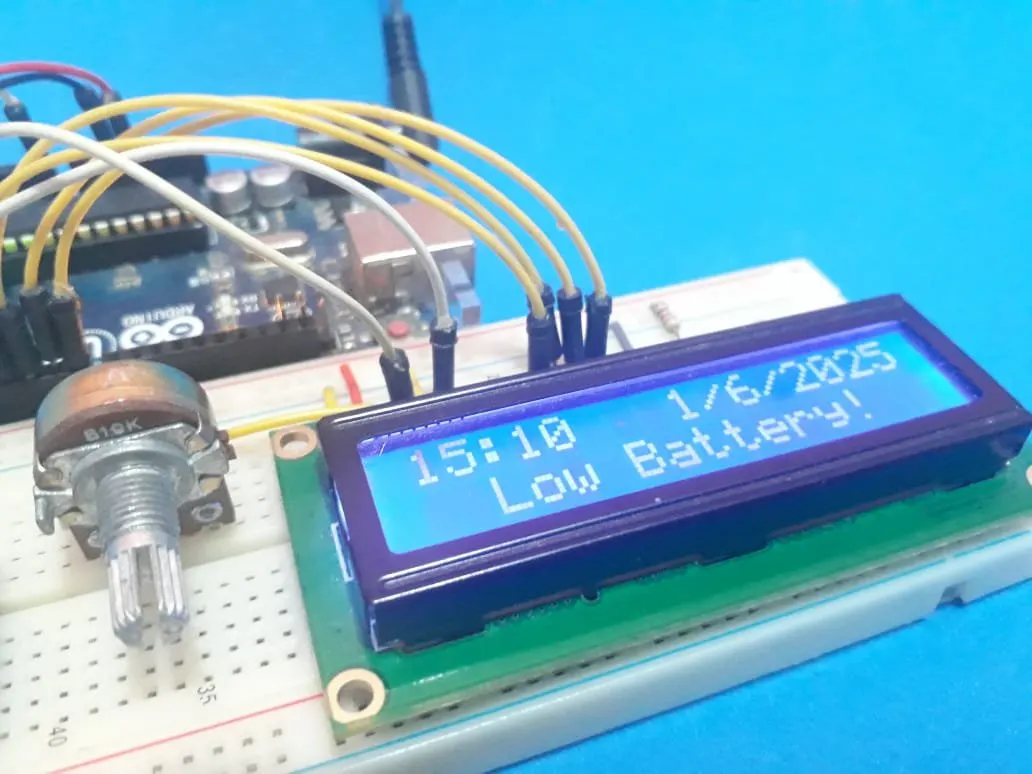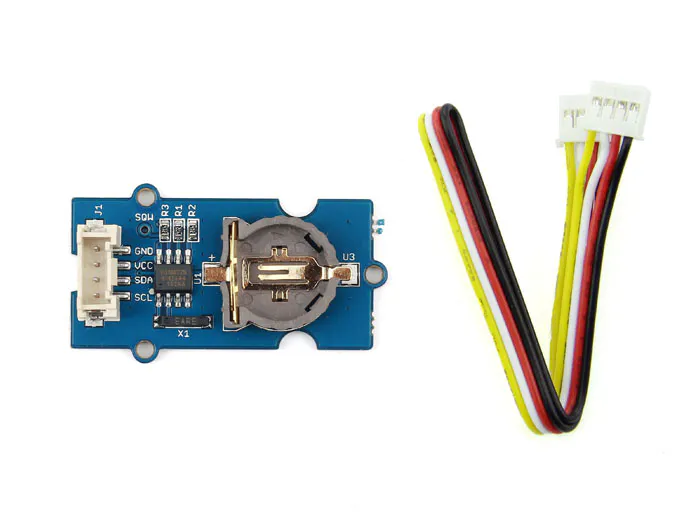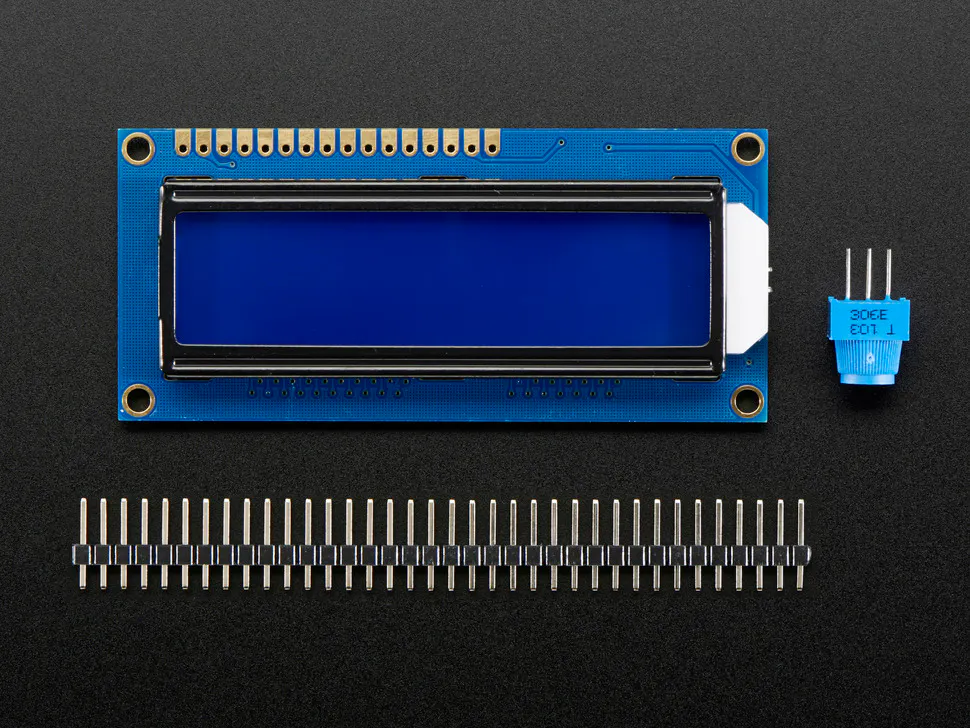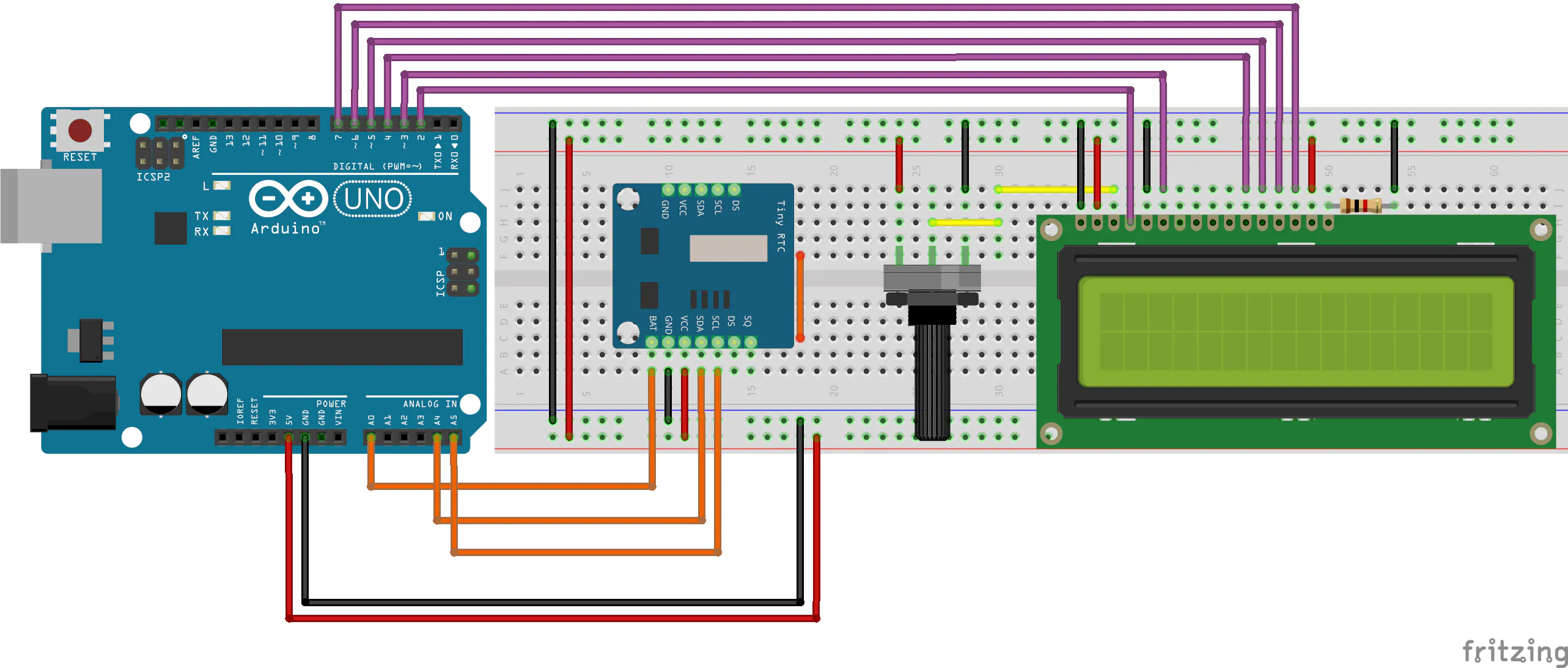Real-Time Clock Battery Monitoring System

In this article, you will learn how to implement a feature to monitor the real-time clock battery and prevent problems in the circuit.

EasyFull instructions provided30 minutes530Things used in this project

Hardware componentsPCBWay Custom PCB
×1Arduino UNO & Genuino UNO
×1Seeed Grove - RTC
×1Adafruit Standard LCD - 16x2 White on Blue
×1

Schematics

Schematic CircuitCode

Battery Voltage Monitor in Real Time Clock

Arduino
```#include <LiquidCrystal.h>
#include <Wire.h>
#include "RTClib.h"

RTC_DS1307 rtc;

const int rs = 2, en = 3, d4 = 4, d5 = 5, d6 = 6, d7 = 7;
LiquidCrystal lcd(rs, en, d4, d5, d6, d7);

#define tensao A0

bool ControlVbat1 = 0, ControlVbat2 = 0;

char WeekDays = {"Domingo", "Segunda", "Terça", "Quarta", "Quinta", "Sexta", "Sábado"};

void setup ()
{
lcd.begin(16,2);
Serial.begin(9600);
if (! rtc.begin())
{
while(1);
}

if (! rtc.isrunning())
{
Serial.println("DS1307 rodando!");
rtc.adjust(DateTime(2025, 6, 1, 15, 00, 00)); //Year, Month, Day, Minutes and Seconds
}

pinMode(tensao, INPUT);
}

void loop ()
{

DateTime now = rtc.now();

lcd.setCursor(0,0);

if(now.hour() < 10)
{
lcd.setCursor(0,0);
lcd.print("0");
}

lcd.print(now.hour(), DEC );
lcd.print(":");

if(now.minute() < 10)
{
lcd.setCursor(3,0);
lcd.print("0");
}

lcd.print(now.minute(), DEC);

lcd.setCursor(8,0);
lcd.print(now.day(), DEC );
lcd.print("/");
lcd.print(now.month(), DEC);
lcd.print("/");
lcd.print(now.year(), DEC);

if(Vbat < 1.5 && ControlVbat1 == 0)
{
lcd.setCursor(0,1);
lcd.print("                ");
lcd.setCursor(2,1);
lcd.print("Low Battery!");
ControlVbat1 = 1;
ControlVbat2 = 0;
}

Serial.println(Vbat);

if(Vbat >= 1.5 && ControlVbat2 == 0)
{
lcd.setCursor(0,1);
lcd.print("                ");
lcd.setCursor(1,1);
lcd.print("Voltage: ");
lcd.print(Vbat);
lcd.print("V");
ControlVbat1 = 0;
ControlVbat2 = 1;
}

}
```

Credits

Silícios Lab

30 projects • 44 followers
Hello, I'm Diego Moreira, founder of the Silícios LAB and I love program microcontrollers and works with electronic projects.

PCBWay

30 projects • 47 followers
We are a pcb prototype and assembly manufacturer to help creators build projects. As low as \$5/10pcs and 3 days delivery time.